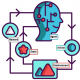###### Reinforcement Q-Learning from Scratch in Python with OpenAI Gym
2019-12-06###### Upper Confidence Bound (UCB) Algorithm Explained with Python code
2019-12-07To answer this question, let’s revisit the components of an MDP, the most typical decision-making framework for RL.

An MDP is typically defined by a 4-tuple (S,A,R,T)( where

S is the state/observation space of an environment
A is the set of actions the agent can choose between
R(s,a) is a function that returns the reward received for taking action a in state s
T(s′|s,a) is a transition probability function, specifying the probability that the environment will transition to state s′ if the agent takes action a in state s.

Our goal is to find a policy ππ that maximizes the expected future (discounted) reward.

Now if we know what all those elements of an MDP are, we can just compute the solution before ever actually executing an action in the environment. In AI, we typically call computing the solution to a decision-making problem before executing an actual decision planning. Some classic planning algorithms for MDPs include Value Iteration, Policy Iteration, and whole lot more.

But the RL problem isn’t so kind to us. What makes a problem an RL problem, rather than a planning problem, is the agent does *not* know all the elements of the MDP, precluding it from being able to plan a solution. Specifically, the agent does not know how the world will change in response to its actions (the transition function T), nor what immediate reward it will receive for doing so (the reward function R). The agent will simply have to try taking actions in the environment, observe what happens, and somehow, find a good policy from doing so.

So, if the agent does not know the transition function T nor the reward function R, preventing it from planning a solution out, how can it find a good policy? Well, it turns out there are lots of ways!

One approach that might immediately strike you, after framing the problem like this, is for the agent to learn a model of how the environment works from its observations and then plan a solution using that model. That is, if the agent is currently in state s1, takes action a1, and then observes the environment transition to state s2 with reward r2, that information can be used to improve its estimate of T(s2|s1,a1) and R(s1,a1), which can be performed using supervised learning approaches. Once the agent has adequately modelled the environment, it can use a planning algorithm with its learned model to find a policy. RL solutions that follow this framework are model-based RL algorithms.

As it turns out though, we don’t have to learn a model of the environment to find a good policy. One of the most classic examples is Q-learning, which directly estimates the optimal Q-values of each action in each state (roughly, the utility of each action in each state), from which a policy may be derived by choosing the action with the highest Q-value in the current state. Actor-critic and policy search methods directly search over policy space to find policies that result in better reward from the environment. Because these approaches do not learn a model of the environment they are called model-free algorithms.

So if you want a way to check if an RL algorithm is model-based or model-free, ask yourself this question: after learning, can the agent make predictions about what the next state and reward will be before it takes each action? If it can, then it’s a model-based RL algorithm. if it cannot, it’s a model-free algorithm.

This same idea may also apply to decision-making processes other than MDPs.##### Amir Masoud Sefidian
Machine Learning Engineer